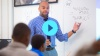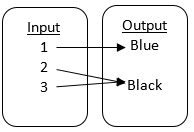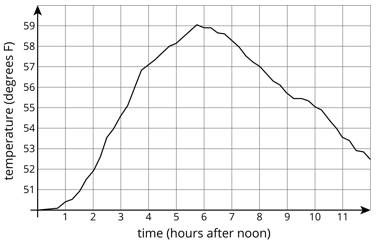# Functions

Students learn how to represent, interpret, and analyze functions in various forms, leading to understanding features such as rates of change, initial values, and intervals of increase and decrease.

Math

Unit 4

## Unit Summary

In Unit 4, 8th grade students are introduced to the concept of a function that relates inputs and outputs. They begin by investigating all types of relationships between sets, such as students and their number of siblings, coins and the number of minutes of parking at a meter, distance and time spent running, etc. They learn how to represent and interpret functions in various forms, including tables, equations, graphs, and verbal descriptions (MP.2). As students progress through the unit, they analyze functions to better understand features such as rates of change, initial values, and intervals of increase or decrease, which in turn enables students to make comparisons across functions even when they are not represented in the same format. Students analyze real-world situations for rates of change and initial values and use these features to construct equations to model the function relationships (MP.4). Students also spend time comparing linear functions to nonlinear functions, building an understanding of the underlying structure of a function that makes it linear (MP.7), setting them up for Unit 5. Lastly, students make connections between stories and graphs by modeling situations like distance or speed over time.

In 6th grade and 7th grade, students studied rate and constant of proportionality in proportional relationships. They developed an understanding of how one quantity changes in relationship to another. Students draw on that knowledge as they investigate how quantities are related in tables, equations, and graphs, and as they investigate linear vs. nonlinear relationships.

Immediately following this unit, students will begin a unit on linear relationships. In that unit, they will revisit and extend on many of the topics introduced in this Functions unit. Students will interpret rate of change as slope and initial value as the $$y-$$intercept of a linear equation $$y=mx+b$$. In high school, the study of functions extends across multiple topics and fields of study, including quadratic, exponential, and trigonometric functions.

Pacing: 16 instructional days (12 lessons, 3 flex days, 1 assessment day)

Fishtank Plus for Math

Unlock features to optimize your prep time, plan engaging lessons, and monitor student progress.## Assessment

The following assessments accompany Unit 4.

### Pre-Unit

Have students complete the Pre-Unit Assessment and Pre-Unit Student Self-Assessment before starting the unit. Use the Pre-Unit Assessment Analysis Guide to identify gaps in foundational understanding and map out a plan for learning acceleration throughout the unit.

### Mid-Unit

Have students complete the Mid-Unit Assessment.

### Post-Unit

Use the resources below to assess student understanding of the unit content and action plan for future units.

Expanded Assessment Package

Use student data to drive your planning with an expanded suite of unit assessments to help gauge students’ facility with foundational skills and concepts, as well as their progress with unit content.

## Unit Prep

### Intellectual Prep

Unit Launch

Prepare to teach this unit by immersing yourself in the standards, big ideas, and connections to prior and future content. Unit Launches include a series of short videos, targeted readings, and opportunities for action planning.#### Internalization of Standards via the Post-Unit Assessment

• Take the Post-Unit Assessment. Annotate for:
• Standards that each question aligns to
• Strategies and representations used in daily lessons
• Relationship to Essential Understandings of unit
• Lesson(s) that Assessment points to

#### Internalization of Trajectory of Unit

• Read and annotate the Unit Summary.
• Notice the progression of concepts through the unit using the Lesson Map.
• Essential Understandings
• Connection to Post-Unit Assessment questions
• Identify key opportunities to engage students in academic discourse. Read through our Teacher Tool on Academic Discourse and refer back to it throughout the unit.

#### Unit-Specific Intellectual Prep

 Model Example Input/output table of functionsEquation of function Degrees Fahrenheit is a function of degrees Celsius $$F=\frac{9}{5}C+32$$ Graph of function Temperature is a function of time.Verbal representation of function The total distance a runner has traveled is a function of time spent running.

### Essential Understandings

• A function is a rule that assigns to each input exactly one output. Functions can be represented as tables, equations, graphs, and verbal descriptions.
• Linear functions consist of ordered pairs that when graphed lie on a straight line; nonlinear functions consist of ordered pairs that when graphed do not lie on a straight line.
• Functions can be analyzed to understand their rate of change, their initial value, or intervals where they may be increasing or decreasing, linear or nonlinear.
• Functions can be used to model relationships between values and shown as equations, graphs, tables, or qualitative descriptions.

### Materials

• Optional: Dry erase marker (1 per student)
• Optional: White board (1 per student)

To see all the materials needed for this course, view our 8th Grade Course Material Overview.

### Vocabulary

function

input/output

initial value

linear function

nonlinear function

rate of change

To see all the vocabulary for Unit 4, view our 8th Grade Vocabulary Glossary.

## Lesson Map

Topic A: Defining Functions

Topic B: Representing and Interpreting Functions

Topic C: Comparing Functions

Topic D: Describing and Drawing Graphs of Functions

## Common Core Standards

Key

Major Cluster

Supporting Cluster

### Core Standards

#### Functions

• 8.F.A.1 — Understand that a function is a rule that assigns to each input exactly one output. The graph of a function is the set of ordered pairs consisting of an input and the corresponding output. Function notation is not required in Grade 8.
• 8.F.A.2 — Compare properties of two functions each represented in a different way (algebraically, graphically, numerically in tables, or by verbal descriptions). For example, given a linear function represented by a table of values and a linear function represented by an algebraic expression, determine which function has the greater rate of change.
• 8.F.A.3 — Interpret the equation y = mx + b as defining a linear function, whose graph is a straight line; give examples of functions that are not linear. For example, the function A = s² giving the area of a square as a function of its side length is not linear because its graph contains the points (1,1), (2,4) and (3,9), which are not on a straight line.
• 8.F.B.4 — Construct a function to model a linear relationship between two quantities. Determine the rate of change and initial value of the function from a description of a relationship or from two (x, y) values, including reading these from a table or from a graph. Interpret the rate of change and initial value of a linear function in terms of the situation it models, and in terms of its graph or a table of values.
• 8.F.B.5 — Describe qualitatively the functional relationship between two quantities by analyzing a graph (e.g., where the function is increasing or decreasing, linear or nonlinear). Sketch a graph that exhibits the qualitative features of a function that has been described verbally.

• 6.EE.A.2.C
• 7.EE.B.4

• 6.RP.A.2
• 7.RP.A.2
• 7.RP.A.2.B
• 7.RP.A.2.C
• 7.RP.A.2.D

• F.BF.A.1

• 8.EE.B.5
• 8.EE.B.6

• F.IF.A.1
• F.IF.A.2
• F.IF.B.4

### Standards for Mathematical Practice

• CCSS.MATH.PRACTICE.MP1 — Make sense of problems and persevere in solving them.

• CCSS.MATH.PRACTICE.MP2 — Reason abstractly and quantitatively.

• CCSS.MATH.PRACTICE.MP3 — Construct viable arguments and critique the reasoning of others.

• CCSS.MATH.PRACTICE.MP4 — Model with mathematics.

• CCSS.MATH.PRACTICE.MP5 — Use appropriate tools strategically.

• CCSS.MATH.PRACTICE.MP6 — Attend to precision.

• CCSS.MATH.PRACTICE.MP7 — Look for and make use of structure.

• CCSS.MATH.PRACTICE.MP8 — Look for and express regularity in repeated reasoning.

Unit 3

Transformations and Angle Relationships

Unit 5

Linear Relationships

## Request a Demo

See all of the features of Fishtank in action and begin the conversation about adoption.

Yes

No

### Any other information you would like to provide about your school?